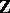# Pseudo-manifold-dimensional and closed (or with boundary)

A finite simplicial complex with the following properties:

a) it is non-branching: Each-dimensional simplex is a face of precisely two (one or two, respectively)-dimensional simplices;

b) it is strongly connected: Any two-dimensional simplices can be joined by a "chain" of-dimensional simplices in which each pair of neighbouring simplices have a common-dimensional face;

c) it has dimensional homogeneity: Each simplex is a face of some-dimensional simplex.

If a certain triangulation of a topological space is a pseudo-manifold, then any of its triangulations is a pseudo-manifold. Therefore one can talk about the property of a topological space being (or not being) a pseudo-manifold.

Examples of pseudo-manifolds: triangulable, compact connected homology manifolds over(cf. Homology manifold); complex algebraic varieties (even with singularities); and Thom spaces (cf. Thom space) of vector bundles over triangulable compact manifolds. Intuitively a pseudo-manifold can be considered as a combinatorial realization of the general idea of a manifold with singularities, the latter forming a set of codimension two. The concepts of orientability, orientation and degree of a mapping make sense for pseudo-manifolds and moreover, within the combinatorial approach, pseudo-manifolds form the natural domain of definition for these concepts (especially as, formally, the definition of a pseudo-manifold is simpler than the definition of a combinatorial manifold). Cycles in a manifold can in a certain sense be realized by means of pseudo-manifolds (see Steenrod problem).